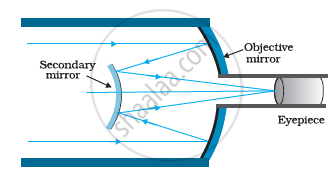Share

# A Cassegrain Telescope Uses Two Mirrors as Shown in Fig. 9.33. Such a Telescope is Built with the Mirrors 20 Mm Apart. If the Radius of Curvature of the Large Mirror is 220 Mm and the Small Mirror is 140 Mm, Where Will the Final Image of an Object at Infinity Be? - CBSE (Science) Class 12 - Physics

#### Question

A Cassegrain telescope uses two mirrors as shown in Fig. 9.33. Such a telescope is built with the mirrors 20 mm apart. If the radius of curvature of the large mirror is 220 mm and the small mirror is 140 mm, where will the final image of an object at infinity be?

#### Solution

The following figure shows a Cassegrain telescope consisting of a concave mirror and a convex mirror.Distance between the objective mirror and the secondary mirror, d = 20 mm

Radius of curvature of the objective mirror, R1 = 220 mm

Hence, focal length of the objective mirror, f_1 = R_1/2 = 110 mm

Radius of curvature of the secondary mirror, R= 140 mm

Hence, focal length of the secondary mirror, f_2 = R_2/2 = 140/2 = 70 min

The image of an object placed at infinity, formed by the objective mirror, will act as a virtual object for the secondary mirror.

Hence, the virtual object distance for the secondary mirror, u = f_1 -d

= 110 -  20

= 90 mm

Applying the mirror formula for the secondary mirror, we can calculate image distance (v)as:

1/v + 1/v = 1/f_2

1/v = 1/f_2 - 1/u

= 1/70 - 1/90 = (9-7)/630 = 2/630

:. v = 630/2 = 315 mm

Hence, the final image will be formed 315 mm away from the secondary mirror.

Is there an error in this question or solution?

#### APPEARS IN

NCERT Solution for Physics Textbook for Class 12 (2018 to Current)
Chapter 9: Ray Optics and Optical Instruments
Q: 36 | Page no. 349
Solution A Cassegrain Telescope Uses Two Mirrors as Shown in Fig. 9.33. Such a Telescope is Built with the Mirrors 20 Mm Apart. If the Radius of Curvature of the Large Mirror is 220 Mm and the Small Mirror is 140 Mm, Where Will the Final Image of an Object at Infinity Be? Concept: Optical Instruments - The Eye.
S